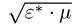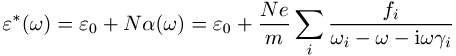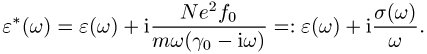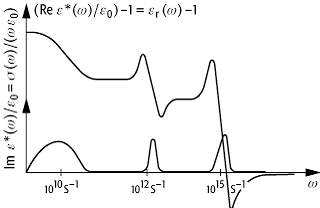# What is the complex dielectric constant

## Techniklexikon.net

Technology lexiconElectrodynamics and Electrical Engineering, e*, generalized, frequency-dependent dielectric constant. The simple approach D = eE. (D: dielectric displacement, E: electric field strength) for the dielectric constant = er / e0 (e0, er: Dielectric constant in a vacuum or in the medium under consideration) applies to fields that vary over time only for individual frequency components in the Fourier expansion: D (w) = e (w) E (w). For a plane wave E (x, t) = ei (k×x-wt) in the non-conductive dielectric then follows from the fourth Maxwell equation, generalized to conductive media:

rotH = ¶D / ¶t + j = -e (w) ¶E / ¶t + s (w) E (1), k´H = -w (w) + is (w) / w) E =: -e*(w) E (2),

with e*(w) = e (w) + is (w) / w (3)

as a general, frequency-dependent and complex dielectric constant in any (isotropic) media (H: magnetic field strength, s (w): electrical conductivity, j: current density). The phase angle of e*(w), d (w), with tand (w) = Ime*(w) / Ree*(w) = we (w) / s (w) also means loss angle and describes a phase shift between E (w) and D (w) that leads to dielectric losses. This phase shift is clearly based on the "lagging" of the reaction of the atomic dipoles to the exciting electrical wave (electrical polarization).

The frequency dependence of e*(w) is explained by the atomic theory of dispersion; e*(w) is with the complex refractive index* via the Maxwell relation n * =connected. With Drude's formula the following applies approximately:(a (w): complex polarizability, N, m: density and mass of the atomic resonators, fi, wi, gi: Oscillator strength, resonance frequency and damping constant of the various resonances). For free electrons in the conductor, e*(w) can be separated into an insulator part e (w) and a conductor part:A comparison with (3) thus results s(w) = f0Ne² / m (g0-iw) = s0 / m (1-iwt), where f0N is the density of conduction electrons, s0 the DC conductivity and t = 1 / g0 = mes0 / Ne² is the relaxation time of the oscillations of the conduction electrons excited by the external field. In the range of the resonance frequencies wi takes Ree*(w) = e (w) strongly from and Ime*(w) becomes maximum (abnormal dispersion). In the other frequency ranges, Ime*(w) »0, e*(w) is real and grows slowly with frequency (normal dispersion). Important resonances are the orientation polarization of permanent dipoles at approx. 1010 Hz. The contribution of the orientation polarization to e*(w) can also be described with the Debye equations. The oscillations of the ions in the crystal lattice are in the infrared spectral range and the polarization of the bound electrons is shifted in the optical and ultraviolet range. In the high frequency limit (hard x-rays and above), e*(w) real again and we have e (w) »1-wPl² / w²<1 (wPl: Plasma frequency). Metals are also "transparent" at these frequencies. This formula also applies to an ideal, collision-free plasma, with collisions e (w) again becomes complex.complex dielectric constant: Schematic representation of the frequency dependence of the complex dielectric constant e*(w).

The free technology lexicon. Well-founded information on all fields of engineering, for scientists, students, practitioners & anyone interested. Professionally presented and accessible free of charge.

Technology lexiconModern physics studies should be made accessible to everyone.